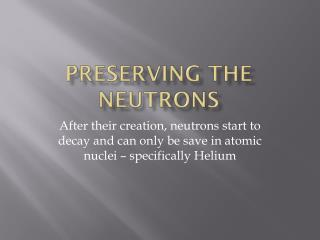DownloadDownload PresentationPreserving the Neutrons

Preserving the Neutrons

Télécharger la présentationPreserving the Neutrons

- - - - - - - - - - - - - - - - - - - - - - - - - - - E N D - - - - - - - - - - - - - - - - - - - - - - - - - - -
Presentation Transcript

1. Preserving the Neutrons After their creation, neutrons start to decay and can only be save in atomic nuclei – specifically Helium

2. Two Unknowns • The Unknown Quark-Gluon Plasma physical behavior • The Unexplained Matter/Anti-Matter Asymmetry  why is it 1 part in 1 billion? • These unknowns are all adequately described in the text material

3. The Thermal History of the Universe • Remember, under conditions of thermal equilibrium all of the physics is determined only by temperature • In general, at early times, this means that the conditions of the Universe at some temperature, T, don’t depend on what happened at earlier times and higher temperature

4. Stage 1: The Universe at 1/100 of a Second: Proton-Neutron Equilibrium: • T = 100 billion K • Density = 1 billion grams per cc  this is below nuclear density so atoms can now exist. • Constituents of the Universe are: • Protons (p+), neutrons (n), electrons (e-) and neutrinos (n) • 1 billion photons for each p+,n,e- • Matter and energy in thermal equilibrium

5. The importance of n • In these two reactions we can see that neutrinos are the mediating agent which allows protons to be converted to neutrons and vice-versa. B = +1 L = +1 C=0 B=1 L=-1 C=+1

6. Proton-Neutron equilibrium • A free neutron will decay in about 900 seconds (its half-life). At t = 0.01 seconds, none of the neutrons have decayed and furthermore, new neutrons are being made from protons. So, as long as the neutrinos can interact with neutrons and protons, this balance will be kept. Hence the proton-to-neutron (p/n) ratio is 1.

7. Stage 2: At 1 Second old. The Neutrons Begin to Decay Away • At this time the Universe has cooled to 10 billion degrees ((still hotter than centers of stars) and the density is around 1000 grams per cubic centimeter. Two important changes are now occurring: • No more proton-anti-proton or neutron-anti-neutron pairs can be created from the radiation field. The average energy per photon is now too low so only light particles (like electrons) can still be produced via pair production.   • The Universe now becomes transparent to neutrinos and thus the neutrinos are no longer interacting with the photons and the neutrons.

8. Stage 3: The Universe from 1-10 seconds: The Last Hurrah for the Electrons • When the Universe is 10 seconds old, it has cooled to the point where the average energy per photon is less than the rest mass energy of any known particle, hence no particles can be created. However, in this interval of 1-10 seconds, the Universe is already below the threshold energy for the creation of P+ and N. • This leads to the important physical situation that the reaction electron+anti-electron --> photon+photonis now greatly favored over the reverse reaction photon+photon --> electron+anti-electron. In fact, it is this electron + anti-electron annihilation that produces most of the photons that we now observe in the CMB. • By t = 10 seconds the (p/n) ratio is now 3:1. No more particle creation is occurring from the photon field. The Universe consists of protons, neutrons and electrons plus a billion photons for each matter particle and non-interacting neutrinos.

9. Stage 5: 3-20 minutes: The Onset of Helium Formation • The Universe has now cooled to a temperature of a few hundred million degrees. The density is approximately 10 grams per cubic centimeter. These values are now very similar to the conditions inside a star. • However, since the Universe is still expanding rapidly, the Universe is not like a star where the temperature and density remain constant. • At this time p/nis 7 and the first steps of the proton-proton cycle begin.

10. The first step • Proton + Proton  goes to 2P bound state but nature doesn’t allow this so one of the P’s turns into a N • So we have proton + proton  (pn) bound state which is called deuterium • Note that the binding energy of (pn) is low and (pn) can easily be photo dissociated back into p + n; the n is now free an can decay

11. Making Helium • H + H ==> 2H (deuterium) + positron + neutrino • 2H + photon ==> p + n  (start over --this is where 1 billion to 1 becomes important) • 2H + H ==> 3He + photon (continue) • 3He + 3He ==> 4He + p + p • where we now have formed stable 4He. If it were not for the formation of 4He, then the Universe would be devoid of neutrons. Thus the free neutrons created in the early Universe end up in 4He nuclei.

12. The whole proton-proton chain

13. Predicted Helium Abundance • The p/n ratio was 7. So for every 14 protons there are 2 neutrons. • The end result of the proton-proton chain is the conversion of 2 protons and 2 neutrons into 1 4He nucleus. • Hence, after the reaction our initial mix of 14 protons and 2 neutrons has been changed to 12 protons and 1 4He nucleus. The mass of 4He is approximately 4 times the mass of a proton. • This leads to a very specific prediction for the mass fraction of the Universe which is in the form of helium: